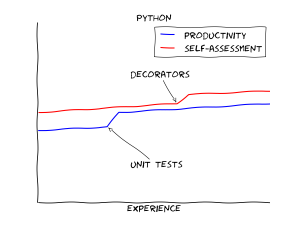# Python装饰器进阶

6860℃

Dobiasd的github上有一篇关于programming_language_learning_curves的文章，其中Python的学习曲线是这样子的。### 1. 增加打印代码

```def argument_print(f):
def helper(x):
print("input:", x)
return f(x)
return helper
@argument_print
def fib(n):
if n == 0:
return 0
elif n == 1:
return 1
else:
return fib(n-1)+fib(n-2)
print(fib(40))
```

### 2. 增加内存缓存

```def memoize(f):
memo = {}
def helper(x):
if x not in memo:
memo[x] = f(x)
return memo[x]
return helper
@memoize
def fib(n):
if n == 0:
return 0
elif n == 1:
return 1
else:
return fib(n-1)+fib(n-2)
print(fib(40))
```

### 3.增加参数检测

```def argument_test_natural_number(f):
def helper(x):
if type(x) == int and x >= 0:
return f(x)
else:
raise Exception("Argument is not an integer")
return helper
@argument_test_natural_number
def fib(n):
if n == 0:
return 0
elif n == 1:
return 1
else:
return fib(n-1)+fib(n-2)
print(fib(40))
```

```@argument_print
@memoize
@argument_test_natural_number
def fib(n):
if n == 0:
return 0
elif n == 1:
return 1
else:
return fib(n-1)+fib(n-2)
print(fib(40))
```